Laplace operator

Assume, we are on the sphere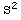with metric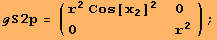φ is an eigenfunction to the Laplace operator on the sphere for any {,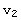,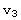}∈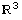\0.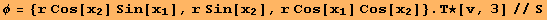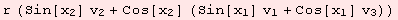The eigenvalue is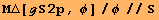The same computation with respect to a different coordinate system is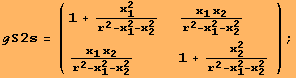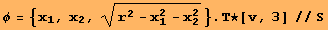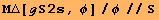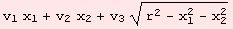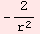The code for the Laplacian is fairly simple: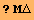Global`Μ△

 Μ△[g_,f_]:=With[{df=Μd[f,ℓ[g]]},ΤC[Inv[g].(Μd[df,1]-df.ΜΓ[g])]]

In the next lines, we set up a parametrization f: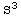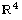of the 3-sphere in, compute the induced metric tensor, and check that φ:R defined below, is an eigenfunction of the Laplace operator.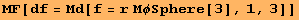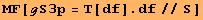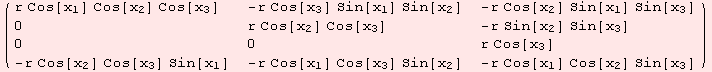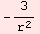Now, back to the. What is the explicit partial differential equation an eigenfunction function of the Laplace operator satisfies on? We give the answer with respect to the two different coordinate systems introduced above.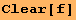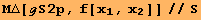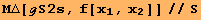Created by Mathematica  (December 22, 2006)